# 基于one-hot编码的词向量方法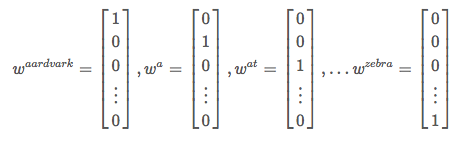one-hot向量将每个单词表示为完全独立的实体，这样的表征方法主要有两个问题：

1. 有序性问题：它无法反映文本的有序性。因为语言并不是一个完全无序的随机序列。比如说，一个字之后只有接特定的字还能组成一个有意义的词，特定的一系列词按特定的顺序组合在一起才能组成一个有意义的句子。
2. 语义鸿沟：其无法通过词向量来衡量相关词之间的距离关系，即这样的表征方法无法反映词之间的相似程度，因为任意两个向量的距离是相同的。

$(w^{hotel})^Tw^{motel} = (w^{hotel})^Tw^{cat} = 0$

1. 维度灾难：高维情形下将导致数据样本稀疏，距离计算困难，这对下游模型的负担是很重的。

# 统计语言模型

$S=w_1, w_2, ... , w_T$

$P(S)=P(w_1, w_2, ..., w_T)=\prod_{t=1}^Tp(w_t|w_1, w_2, ..., w_{t-1})$

$p(w_t|w_1,w_2,...,w_{t-1})$

$p(w_t|w_1, w_2, ..., w_{t-1}) \approx p(w_t|w_{t-n+1}, ..., w_{t-1})$

# 从分布式表征到SVD分解

## 分布式表征（Distribution）

1. 由于参数空间的爆炸式增长，它无法处理更长程的context（N>3）；
2. 其次，它虽然可以描述上下文之间的概率关系，但这种处理方式本质的数学的形式仍是一个个离散的one-hot向量，或者说Ngram仅仅解决了文本之间的转移概率的问题，并没有解决文本本身的表征问题。例如，假设我们在训练语料中看到了很多类似“the dog is walking in the bedroom”或是“the cat is running in the bedroom”这样的句子，那么，即使我们看不懂英文，也可以明白“cat”和“dog”（“walking”和“running”）之间是相似的。然而，Ngram模型能描述的只有running出现在“cat”和“dog”之后的概率都很高，但是无法表述“cat”与“dog”是这两个词是相似的。
3. 我们知道one-hot向量的长度对应于整个词表的长度。在动辄上完甚至上百万的实际应用中，这样的表征方法将面临巨大的维度灾难问题。

## 奇异值分解（SVD）

$Av = \lambda v$

$A = Q \Sigma Q^{-1}$

$A = U \Sigma V^T$

$A = U \Sigma V^T => A^T = V \Sigma U^T => A^TA = V \Sigma U^TU \Sigma V^T = V \Sigma^2 V^T$

$A_{m\times n} \approx U_{m\times r}\Sigma V^T_{r\times n}$

## 基于SVD的词向量方法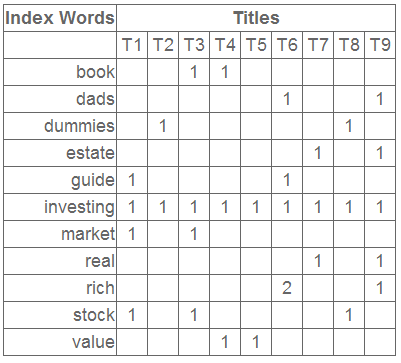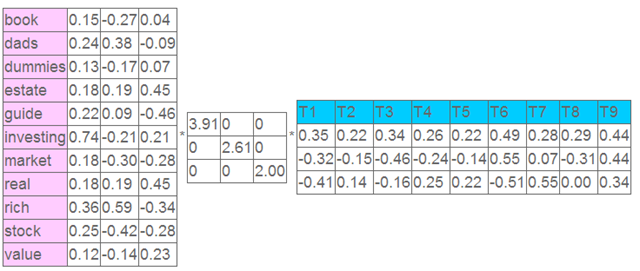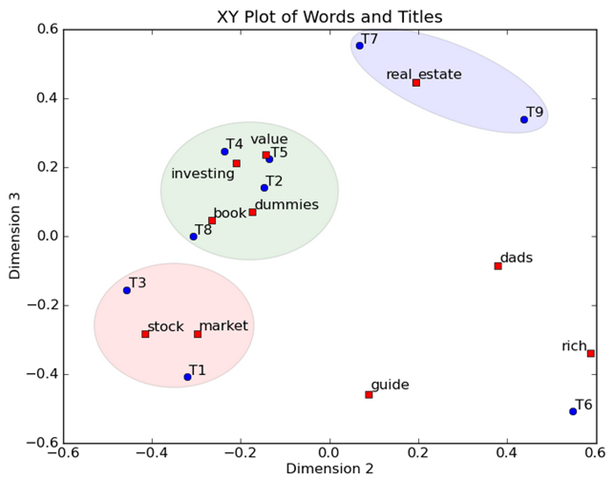# 神经网络语言模型（Neural Network Language Model）

NNLM模型的基本思想可以概括如下：

1. 假定词表中的每一个word都对应着一个连续的特征向量；
2. 假定一个连续平滑的概率模型，输入一段词向量的序列，可以输出这段序列的联合概率；
3. 同时学习词向量的权重和Ngram概率模型里的参数。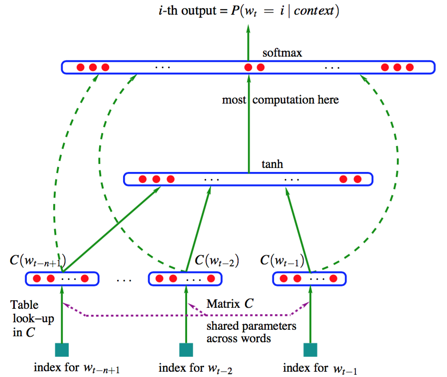• 首先是一个线性的映射层。它将输入的N−1个one-hot词向量，通过一个共享的D×V的矩阵C，映射为N−1个分布式的词向量（distributed vector）。其中，V是词典的大小，D是Embedding向量的维度（一个先验参数）。C矩阵里存储了要学习的word vector，为什么这是我们需要的词向量呢？试想一下，当我们把n个one-hot表征的词向量词典输入到神经网络中，单层的神经网络进行的运算无非就是$Y = W^TX$，这个操作等效于查表操作，one-hot向量将n个词向量从Embedding层中原封不动地提取出来，如下图所示。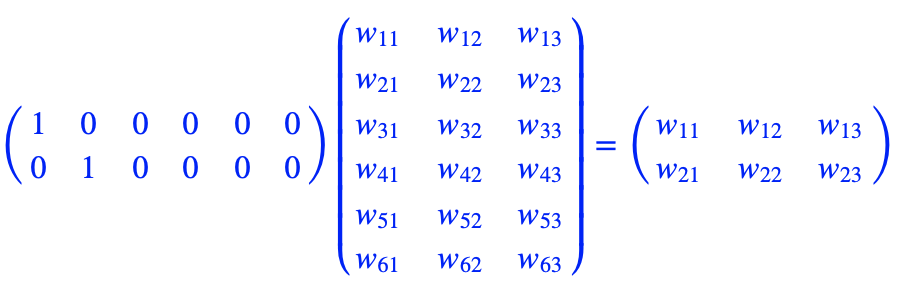这样，我们在训练语言模型的过程中，映射层的权值就等效为我们需要的词向量表征。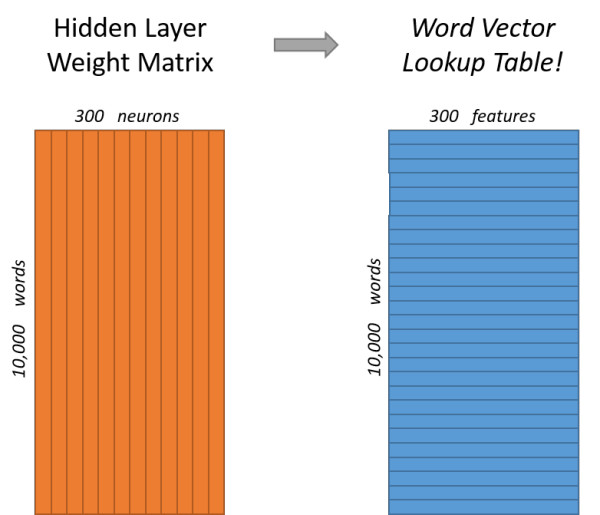• 其次是一个简单的前向反馈神经网络g。它由一个激活函数为tanh的隐藏层和一个softmax输出（分类）层组成，，可以将我们得到的一系列输出映射成对应概率。这样，通过将Embedding层输出的N−1个词向量映射为一个长度为V的概率分布向量，从而对词典中的word在输入context下的条件概率做出预估：

$p(w_i|w_1,w_2,...,w_{t-1}) \approx f(w_i, w_{t-1}, ..., w_{t-n+1}) = g(w_i, C(w_{t-n+1}), ..., C(w_{t-1}))$

$L(\theta)=\frac{1}{T}\sum_t{\log{f(w_t, w_{t-1}, ..., w_{t-n+1})}}+R(\theta)$

1. 一个是统计语言模型里关注的条件概率$p(w_t|context)$的计算；
2. 一个是向量空间模型里关注的词向量的表达。

1. 一个问题是，由于NNLM模型使用的是全连接神经网络，因此只能处理定长的序列。
2. 另一个问题是，由于其巨大的参数空间，将NNLM的训练太慢了。即便是在百万量级的数据集上，即便是借助了40个CPU进行训练，NNLM也需要耗时数周才能给出一个稍微靠谱的解来。显然，对于现在动辄上千万甚至上亿的真实语料库，训练一个NNLM模型几乎是一个impossible mission。

# Word2Vec

1. 用一个简单模型训练出连续的词向量；
2. 基于词向量的表达，训练一个连续的Ngram神经网络模型。

## 两个模型

### CBoW（Continues Bag-of-Words Model）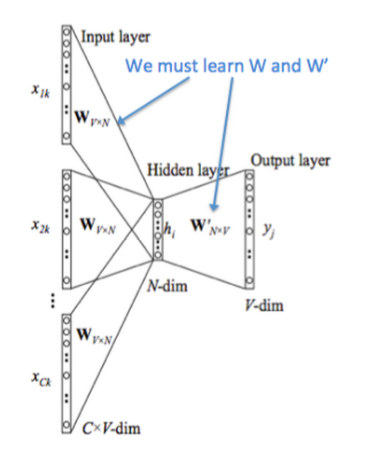• 图中 $[x_{1k}, ..., x_{Ck}]$ 表示第 $k$ 个中心词的前后$C$个上下文的 one-hot 向量
• 将 one-hot 向量输入存放词向量的矩阵 $W_{V\times N}$进行查表，$V$为词表的大小，$N$为词向量的维度
• 将查表得到的上下文向量直接进行求和，再通过一个$N \times V$的矩阵映射到输出层

1. 移除NNLM模型中的Hidden layer结构；
2. 直接将Embedding layer的查表之后累加求和（NNLM是将输出结果拼接）
3. 将下文单词纳入上、下文环境，真正考虑了Context（NNLM的输入严格来说为上文文本）

### Skip-gram

CBoW模型依然是从context对target word的预测中学习到词向量的表达。反过来，我们能否从target word对context的预测中学习到word vector呢？答案显然是可以的：这便是Skip-gram模型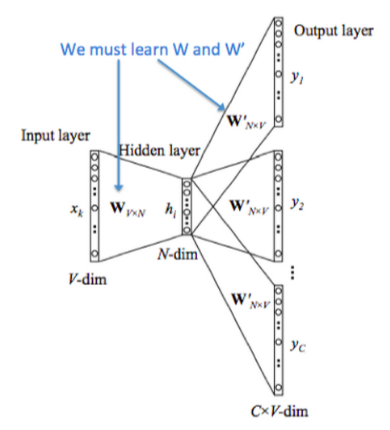$P(w_0|w_i)=\frac{e^{U_0 \cdot V_i}}{\sum_je^{U_j \cdot V_i}}$

Skip-gram模型的本质是计算输入word的input vector与目标word的output vector之间的余弦相似度，并进行softmax归一化。我们要学习的模型参数正是这两类词向量。

## 两个提速手段

### 层次Softmax(Hierarchical Softmax)

Hierarchical Softmax是word2vec中的一项关键技术，简单来说，其通过构造一个Huffman树，将复杂的归一化概率问题转化为一系列二分类的条件概率相乘的形式。首先，我们需要了解什么是Huffman树。

#### Huffman树

Huffman编码又称为最优二叉树，表示一种带权路径长度最短的二叉树。带权路径长度，指的就是叶子结点的权值乘以该结点到根结点的路径长度。而我们需要构造的Huffman树结构，是以词表为根结点，每一个子节点为父节点的不相交子集，词为叶节点的结构。我们将叶节点的权值转化为词频，则带权路径长度指的就是词频乘以路径的大小，带权路径最小的条件使得构造出来的霍夫曼树中，高频词离根结点更近，而低频词离根结点更远。其构造的Huffman树如下所示：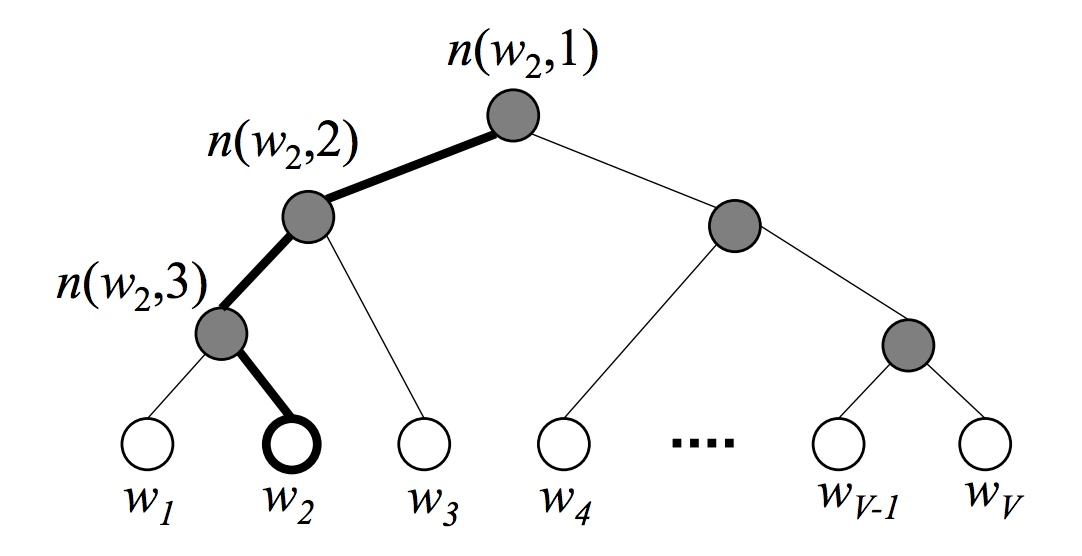#### 目标词概率

$p(w_t \in D_1|context)=\frac{1}{1+e^{-U_{D_{root}} \cdot V_{w_t}}}$

$p(w_t|context)=p(D_1=1|context)p(D_2=0|D_1=1)\dots p(w_t|D_k=1)$

### 负采样(Nagative Sampling)

$J(\theta)=-\frac{1}{T}\sum_{t=1}^T{\sum_{-c \leq j \leq c, j \neq 0}{\log p(w_{t+j}|w_t)}}$

$p(D=1|w, context)=\frac{p(w|context)}{p(w|context)+kp_n(w)}=\sigma (\log p(w|context) - \log kp_n(w))$

1. 首先确定正样本，通过计算中心词与上下文中词的其余弦相似度，再用一个sigmoid函数来判断：

$p(D=1|w_o, w_i)=\sigma (U_o \cdot V_i)$

1. 采样词典中不在中心词上下文中的词的词作为负样本，采样频率由该词在语料库中出现的频率有关，作者给出了一个经验公式：

$P(w_i) = \frac{f(w_i)^{3/4}}{\sum^n_{j=0}(f(w_j)^{3/4})}$

## 一些预处理细节

word2vec的训练是支持多线程的，可以通过设置num_threads来设置使用的线程数。

## word2vec的局限性

1. 在模型训练的过程中仅仅考虑context中的局部语料，没有考虑到全局信息；
2. 对于英文语料，对于什么是词，怎样分词并不是问题（但个词就是独立的个体）。而对于中文而言，我们在训练词向量之前首先要解决分词的问题，而分词的效果在很多情况下将会严重影响词向量的质量（如分词粒度等），因此，从某些方面来说word2vec对中文不是那么的友好；
3. 在2018年以前，对于word2vec及其一系列其他的词向量模型都有一个相同的特点：其embedding矩阵在训练完成后便已经是固定了的，这样我们可以轻易从网上获取到大量预训练好的词向量并快速应用到我们自己任务中。但从另一个角度来说，对于同一个词，在任意一个句子，任意一个环境下的词向量都是固定的，这对于一些歧义词来说是存在较大问题的，这也是限制类似word2vec、Glove等词向量工具性能的一个很重要的问题。

# GloVe

## GloVe的代价函数

$J = \sum_{i,j}^{N}(w_i^Tw_j + b_i + b_j - log(X_{i, j}))^2$

• 单词$k$出现在单词$i$上下文的条件概率：

$P_{i,k}=\frac{X_{i, k}}{\sum_{j=1}^NX_{i,j}}=\frac{X_{i, k}}{X_i}$

• 定义单词$k$出现在两个不同单词$i, j$上下文的两个条件概率的比率：

$ratio_{i, j, k}=\frac{P_{i, k}}{P_{j, k}}$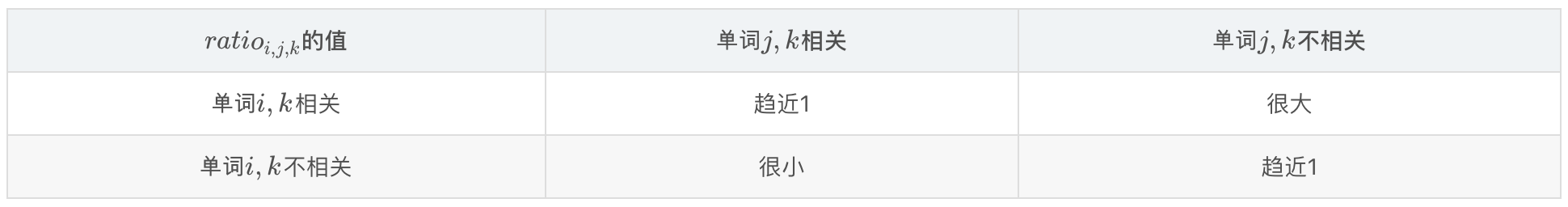$g(w_i,w_j,w_k) =ratio_{i, j, k} = \frac{\hat{P_{i, k}}}{\hat{P_{j, k}}}$

$J = \sum_{i,j,k}^{N}(\frac{P_{i, k}}{P_{j, k}}-g(w_i,w_j,w_k))^2$

$\frac{\hat{P_{i, k}}}{\hat{P_{j, k}}} = exp((w_i-w_j)^Tw_k) = \frac{exp(w_i^Tw_k)}{exp(w_j^Tw_k)}$

$\frac{exp(w_i^Tw_k)}{exp(w_j^Tw_k)} = \frac{\hat{P_{i, k}}}{\hat{P_{j, k}}} --> \frac{P_{i, k}}{P_{j, k}}$

$exp(w_i^Tw_k) = \hat{P_{i, k}} --> P_{i, k}$

$J = \sum_{i,j}^{N}(P_{i, j}-g(w_i,w_j))^2 = \sum_{i,j}^{N}(P_{i, j}-exp(w_i^Tw_j))^2$

$J = \sum_{i,j}^{N}(log(P_{i, j})-w_i^Tw_j)^2$

$log(P_{i, j}) \neq log(P_{j, i})$

$w_i^Tw_j = w_j^Tw_i$

$log(P_{i, j}) = log(X_{i,j})-log(X_i)$

$w_i^Tw_j = log(\hat{P_{i, j}}) --> log(P_{i, j})$

$w_i^Tw_j + b_i + b_j = \hat{X_{i,j}} --> X_{i, j}$

$J = \sum_{i,j}^{N}(w_i^Tw_j + b_i + b_j - log(X_{i, j}))^2$

$J = \sum_{i,j}^{N}f(X_{i,j})(w_i^Tw_j + b_i + b_j - log(X_{i, j}))^2$

$f(x)=\begin{cases} (x/xmax)^{0.75} & x<x_{max} \\ 1 & x \ge x_{max} \end{cases}$

## 小结

GloVe与Word2Vec的区别就在于GloVe考虑到了文本的全局特征，直观上来说比Word2Vec更合理。在语料库足够大的情况下GloVe的效果通常会更好一些，但这也不是绝对，word2vec在很多任务上的表现也比GloVe要更好。可以尝试的做法是将GloVe和Word2Vec进行整合（拼接等操作），会使得模型有意想不到的提升。

posted @ 2019-09-29 15:18  西多士NLP  阅读(5696)  评论(0编辑  收藏  举报